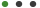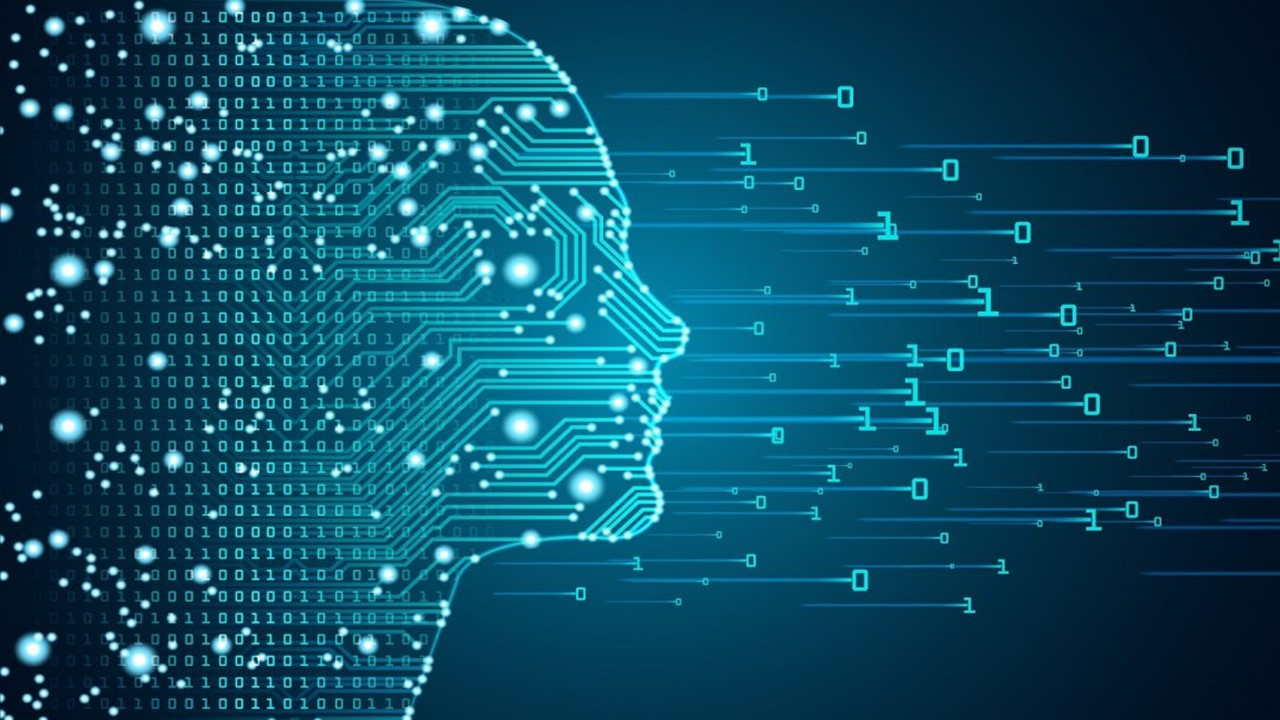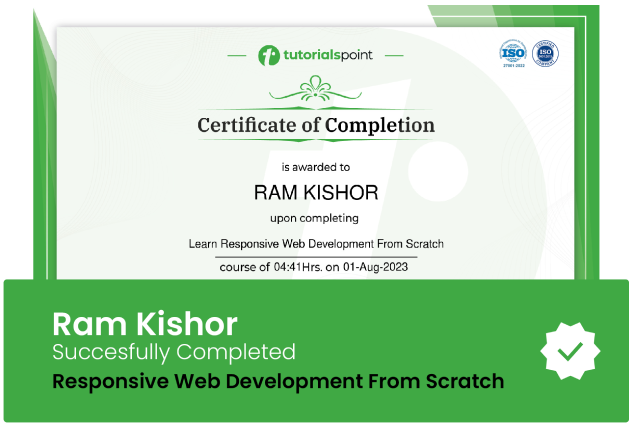### Machine Learning with Python Basics (For Beginners)Mohamed Gamal

# Machine Learning with Python Basics (For Beginners)

Learn the Basics of Machine Learning with Python (First Step For Beginners)Updated on Nov, 2023Language - EnglishMohamed GamalMachine Learning,Data Science,Development

Lectures -51

Resources -2

Duration -5.5 hours30-days Money-Back Guarantee

Training 5 or more people ?

## Course Description

Steps of Machine Learning that you Will learn:

1. Import the data.

2. Split data into Training & Test.

3. Create a Model.

4. Train The Model.

5. Make Predictions.

6. Evaluate and improve.

Machine Learning Course Contents:

1. What is Machine Learning - Types of Machine Learning (Supervised & Unsupervised).

2. Linear Regression with One Variable.

3. Linear Regression with One Variable (Cost Function - Gradient Descent).

4. Linear Regression with Multiple Variable.

5. Logistic Regression (Classification).

6. Logistic Regression (Cost Function - Gradient Descent).

7. Logistic Regression (Multiclass).

8. Regularization Overfitting.

9. Regularization (Linear and Logistic Regression).

10. Neural Network Overview.

11. Neural Network (Cost Function).

12. Advice for Applying Machine Leaning.

13. Machine Learning Project 1

14. Machine Learning Project 2

Python Basics Course Contents:

1. How to print

2. Variables

4. Type Conversion

5. String

6. Formatted String

7. String Methods

8. Arithmetic Operations

9. Math Functions

10. If Statement

11. Logical Operators

12. Comparison Operators

13. While

14. For Loops

15. Nested Loops

16. List

17. 2D List

18. List Methods

19. Tuples

20. Unpacking

21. Dictionaries

22. Functions

23. Parameters

24. Keyword Arguments

25. Return Statement

26. Try - Except

28. Classes

Notes:

1. You will learn the basics of Machine Learning.

2. You will learn the basics of python.

3. You will need to set up Anaconda.

4. You will need to setup python & PyCharm

5. This course is considered the first step in Machine Learning.

7. No Programming Experience is Needed for this course.

8. Python for Data Science and Machine Learning is a great course that you can take to learn the implementation of ML models in Python.

9. This course is considered step one in Machine Leaning, You will learn the concept of Machine Learning with python basics.

### Goals

What will you learn in this course:

• You Will learn basic concept of Machine Learning, Types of Machine Learning
• You Will learn basic concept of Linear Regression With One Variable & Multiple
• You Will learn basic concept of Logistic Regression
• You Will learn basic concept of Regularization (Linear and Logistic Regression)
• You Will learn basic concept of Neural Network
• Machine Learning Projects

### Prerequisites

What are the prerequisites for this course?

• No Programming Experience Needed## Curriculum

Check out the detailed breakdown of what’s inside the course

Introduction
1 Lectures
•Introduction 01:54 01:54
Machine Learning with Python Basics
13 LecturesMachine Learning Projects
3 LecturesPython Basics
31 LecturesPython Projects
3 Lectures## Instructor DetailsMohamed Gamal

Have strong experience as CAD CAM Instructor:

NX (CAD - CAM - CAE -Mold Wizard - CNC Post Builder, Sheet Metal)

Solid Works, Inventor,  Solid Edge

Have strong experience in post processing for CNC machines (Milling, Turning, Wire EDM)

Making a (G code) programs for a lot of mechanical products and manufacturing it in CNC machines (Milling 3 Axis, Multi Axis).

Have strong knowledge of mold wizard of NX (Injection Mold Plastic )

Making a complete mold base for plastic parts and get the drawings for the manufacturing.

## Course Certificate

User your certification to make a career change or to advance in your current career. Salaries are among the highest in the world.